## 2.5.1 Phonon Dispersion Relations of Graphene

Since there are two carbon atoms,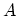and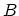, in the unit cell of graphene, one must consider 6 coordinates. The secular equation to be solved is thus a dynamical matrix of rank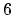, such that 6 phonon branches are achieved.

The phonon dispersion relation of the graphene comprises three acoustic (A) branches and three optical (O) branches. The modes are associated with out-of-plane (Z), in-plane longitudinal (L), and in-plane transverse (T) atomic motions (Fig. 2.10-a).

Figure 2.10-b shows the phonon dispersion branches of graphene. The three phonon dispersion branches, which originate from the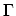-point of the BRILLOUIN zone correspond to acoustic modes: an out-of plane mode (ZA), an in-plane transverse mode (TA), and in-plane longitudinal (LA), listed in order of increasing energy. The remaining three branches correspond to optical modes: one out-of plane mode (ZO), and two in-plane modes (TO) and (LO).

While the TA and LA modes display the normal linear dispersion around the-point, the ZA mode shows a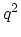energy dispersion which is explained in  as a consequence of the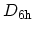point-group symmetry of graphene. Another consequence of the symmetry are the linear crossings of the ZA/ZO and the LA/LO modes at the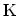-point.

Figure 2.10: a) Atomic motions of carbon atoms in graphence can be along the out-of-plane (Z), in-plane transverse (T), and in-plane longitudinal (L) direction. b) The phonon dispersion branches for a graphene sheet, plotted along high symmetry directions .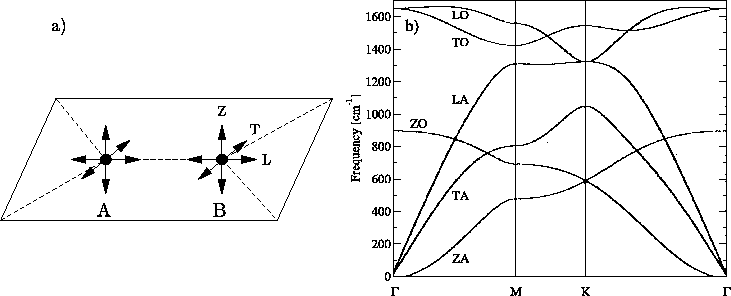M. Pourfath: Numerical Study of Quantum Transport in Carbon Nanotube-Based Transistors